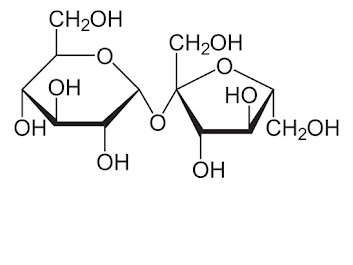plant biochem final quiz 2019
Last Name *
First Name *
period *
1. Please refer to the diagram below. If the entire chain is a polymer, each circle is a __________ and the lines between each circle represents a ___________.
2 points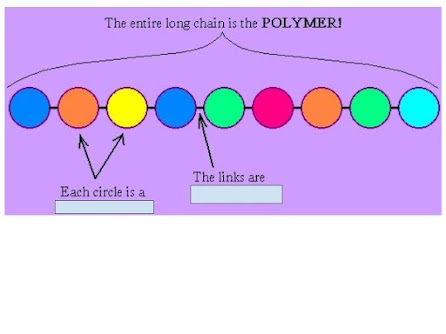2. The molecule below is a short protein. . How many molecules of water were "removed" in the process of dehydration synthesis to make it?
2 points3. Which choice matches the POLYMER with its correct MONOMER
2 points
4. What would be the molecular formula for a monosacharide called alpha glucose?
2 points
5. Which of the following categories of organic molecules is correctly paired with one of its functions
2 points
6. What would be the molecular formula for a monosacharide called fructose?
2 points
7. Which of the following terms includes all the other terms on this list?
2 points
8. Which of the following is NOT associated with proteins (check all that apply)
2 points
9. How many different amino acids are used in making plant proteins?
2 points
10. Which part of an amino acid are ALWAYS the same. Select all that apply
2 points
11. Please refer to diagram 2 below. Letter A is pointing to
2 points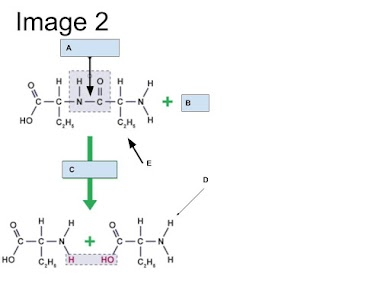12. Please refer to diagram 2 below. Letter D is pointing to
2 points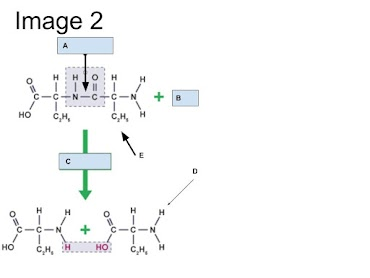13. All of the following are examples of lipids EXCEPT
2 points
14. Figure 1 below represents a molecule most likely derived from a(n)
2 points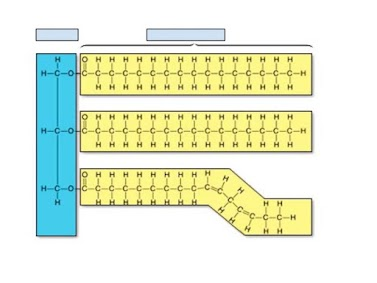15. The molecule in the figure 1 below was synthesized by attaching the molecule highlighted in blue to the yellow molecules. What are the name of the molecules highlighted in yellow?
2 points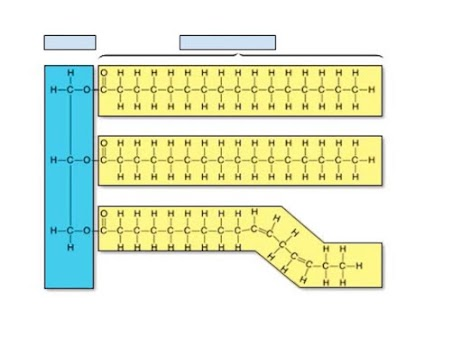16. A major type of lipid found in the cell membranes of plants are:
2 points
17. Lipids serve a number of functions in living organisms. Which of the following is NOT a function of lipids
2 points
18. The diagram below is of a simple sugar called ___________.
2 points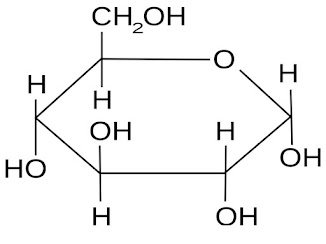19. If we assembled 1000's of the molecule below, I would have made (check all that apply)
2 points19. The molecule below is called a _________. Check all that apply
2 points